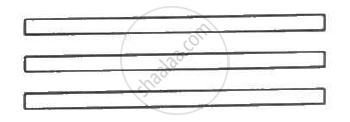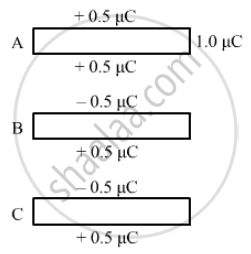Department of Pre-University Education, Karnataka course PUC Karnataka Science Class 12
Share

# The Capacitance Between the Adjacent Plates Shown in the Figure is 50 Nf. a Charge of 1.0µC is Placed on the Middle Plate. - Physics

ConceptElectric Potential Difference

#### Question

The capacitance between the adjacent plates shown in the figure is 50 nF. A charge of 1.0µC is placed on the middle plate. If 1.0 µC is placed on the upper plate instead of the middle, what will be the potential difference between (a) the upper and the middle plates and (b) the middle and the lower plates?#### SolutionWhen 1µC charge is given to the upper plate, the charge gets distributed. The two sides of the upper plate have +0.5  muC charge. One side of the middle plate facing the upper plate has -0.5  muC charge and the other side has +0.5  muC Change . Similarly, one side of the lower plate facing the middle plate has -0.5  muC charge and the other side has +0.5  muC charge.

(a) Effective charge on the capacitor formed by the upper and middle plates = 0.5 µC

Capacitance = 50 nF = 0.05 µF

∴ Potential difference between the plates , V = Q/C = (0.5  muC)/(0.05  muF) = 10 "V"

(b) Effective charge on the capacitor formed by the middle and lower plates = 0.5 µC

Capacitance = 0.50 µF

∴ Potential difference between the plates, V = Q/C = (0.5  muC)/(0.05  muF) = 10 "V"

Is there an error in this question or solution?

#### Video TutorialsVIEW ALL 

Solution The Capacitance Between the Adjacent Plates Shown in the Figure is 50 Nf. a Charge of 1.0µC is Placed on the Middle Plate. Concept: Electric Potential Difference.
S